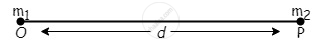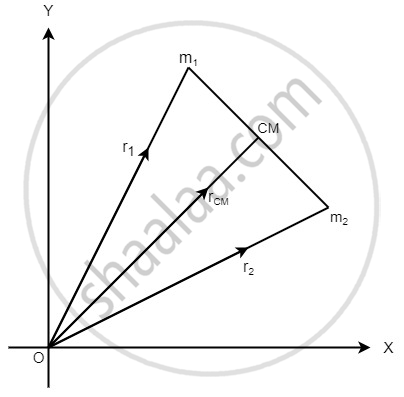# Centre of Mass

### Centre of Mass:

Centre of mass of a system is the point that behaves as whole mass of the system is concentrated at it and all external forces are acting on it.

For rigid bodies, centre of mass is independent of the state of the body i.e., whether it is in rest or in accelerated motion centre of mass will remain the same.

### Centre of Mass of System of n Particles

If a system consists of n particles of masses m1, m2, m3,… mn having position vectors rl, r2, r3,… rn. then position vector of centre of mass of the system, "r"_("CM") = ("m"_1"r"_1 + "m"_2"r"_2 + "m"_3"r"_3 + ... + "m"_"n" "r"_"n")/("m"_1 + "m"_2 + "m"_3 + ... + "m"_"n") =(sum_(i = 1)^"n" "m"_"i" "r"_"i")/(sum "m"_"i")

In terms of coordinates,

x_("CM") = ("m"_1x_1 + "m"_2x_2 + ... +"m"_"n" x_"n")/("m"_1 + "m"_2 + ... +"m"_"n") = (sum_(i = 1)^"n" "m"_ix_i)/(sum "m"_i)

"y"_("CM") = ("m"_1"y"_1 + "m"_2"y"_2 + ... +"m"_"n" "y"_"n")/("m"_1 + "m"_2 + ... +"m"_"n") = (sum_(i = 1)^"n" "m"_i"y"_i)/(sum "m"_i)

"z"_("CM") = ("m"_1"z"_1 + "m"_2"z"_2 + ... +"m"_"n" "z"_"n")/("m"_1 + "m"_2 + ... +"m"_"n") = (sum_(i = 1)^"n" "m"_i"z"_i)/(sum "m"_i)

Centre of Mass of Two Particle System

Choosing O as origin of the coordinate axis.

(i) Then, position of centre of mass from "m"_1 = ("m"_2"d")/("m"_1 + "m"_2)(ii) Position of centre of mass from "m"_2 = ("m"_1"d")/("m"_1 +"m"_2)

(iii) If position vectors of particles of masses m1 and m2 are r1 and r2respectively, then

r_(CM) = ("m"_1"r"_1 + "m"_2"r"_2)/("m"_1 +"m"_2)(iv) If in a two particle system, particles of masses m1 and m2 moving with velocities v1 and v2 respectively, then velocity the centre of mass.

"V"_("CM") = ("m"_1"v"_1 + "m"_2"v"_2)/("m"_1 +"m"_2)

(v) If accelerations of the particles are a1, and a2 respectively, then acceleration of the centre of mass.

a_(CM) = ("m"_1"a"_1 + "m"_2"a"_1)/("m"_1 + "m"_2)

(vi) Centre of mass of an isolated system has a constant velocity.

(vii) It means isolated system will remain at rest if it is initially rest or will move with a same velocity if it is in motion initially.

(viii) The position of centre of mass depends upon the shape, size and distribution of the mass of the body.

(ix) The centre of mass of an object need not to lie with in the object.

(x) In symmetrical bodies having homogeneous distribution mass the centre of mass coincides with the geometrical centre the body.

(xi) The position of centre of mass of an object changes translational motion but remains unchanged in rotatory motion.

If you would like to contribute notes or other learning material, please submit them using the button below.